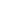Weekly Boost

Week 5Multiplication and Mixed Practice

Learners will practice correctly solving multi-digit multiplication problems with decimals. Then, they'll read a variety of sentences, determine which of the sentences use similes and which use metaphors, and write out the literal meanings.
Students will practice correctly solving multi-digit multiplication problems with decimals. Then, they'll read a variety of sentences, determine which of the sentences use similes and which use metaphors, and write out the literal meanings.
Dizzy Over Decimals: Multiplying Decimals #1
Worksheet
Dizzy Over Decimals: Multiplying Decimals #1
Learners practice multiplying multi-digit numbers with decimals in this playful sixth- and seventh-grade math worksheet.
Math
Worksheet
Mixed Practice: Similes and Metaphors #1
Worksheet
Mixed Practice: Similes and Metaphors #1
Children work with two forms of figurative language in this grammar worksheet.
WorksheetSend home these fun free resources to help reinforce the skills you're teaching in the classroom.